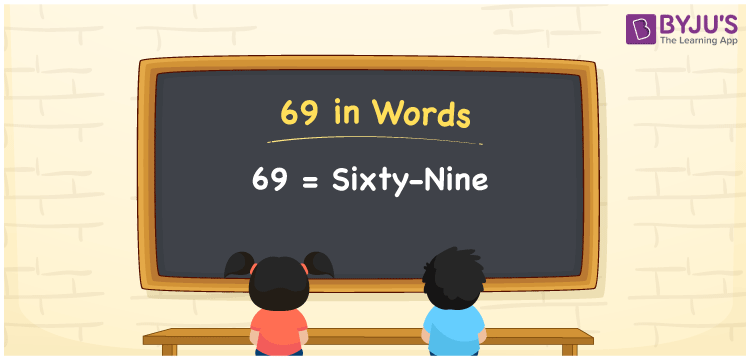# 69 in Words

69 in words is written as “Sixty-nine”. 69 is 5 more than 40. In the number system, 69 is a number that represents a value or a count equivalent to it. For example, Samrah has bought a book for Rs.69. Using words, we can write the same sentence as Samrah has bought a book for Rupees Sixty-nine. Thus, we can have many real-life examples where the numbers are presented in words. Thus it is necessary for students to learn the number names to read, write and express them. Let us learn how to write 69 in words in this article.

 69 in words Sixty-nine Sixty-nine in Numbers 69

## 69 in English Words

We generally write numbers in words using the English alphabet. Thus, we can read 69 in English as “Sixty-nine”.## How to Write 69 in Words?

To convert 69 in words, we need to check the place value of its digits. Since 69 is a two-digit number, thus, place value of 6 is tens and 9 is ones. The order of place values of digits in a number is in accordance with the Indian numbering system, such as:

• Ones
• Tens
• Hundreds
• Thousands
• Ten-thousands
• Hundred-thousands or Lakhs

This can be done as follows.

 Tens Ones 6 9

This can be written as:

6 × Ten + 9 × One

= 6 × 10 + 9 × 1

= 60 + 9

= 69

= Sixty-nine

Thus, 69 in words is written as Sixty-nine.

69 is a natural number that precedes 70 and succeeds 68.

69 in words – Sixty-nine

Is 69 an odd number? – Yes

Is 69 an even number? – No

Is 69 a perfect square number? – No

Is 69 a perfect cube number? – No

Is 69 a prime number? – No

Is 69 a composite number? – Yes

## Frequently Asked Questions on 69 in Words

Q1

### How do you write 69 in English?

We can write 69 in English words as “Sixty-nine”.
Q2

### What is 169 in English?

169 is written as One hundred and Sixty-nine in English.
Q3

### Is 69 an even number or an odd number?

69 is an odd number since it is not completely divisible by 2.